Fitting Linear Models to Data

LEARNING OBJECTIVES

By the end of this lesson, you will be able to:

• Draw and interpret scatter plots.
• Find the line of best fit.
• Distinguish between linear and nonlinear relations.
• Use a linear model to make predictions.

A professor is attempting to identify trends among final exam scores. His class has a mixture of students, so he wonders if there is any relationship between age and final exam scores. One way for him to analyze the scores is by creating a diagram that relates the age of each student to the exam score received. In this section, we will examine one such diagram known as a scatter plot.

Drawing and Interpreting Scatter Plots

A scatter plot is a graph of plotted points that may show a relationship between two sets of data. If the relationship is from a linear model, or a model that is nearly linear, the professor can draw conclusions using his knowledge of linear functions. Below is a sample scatter plot.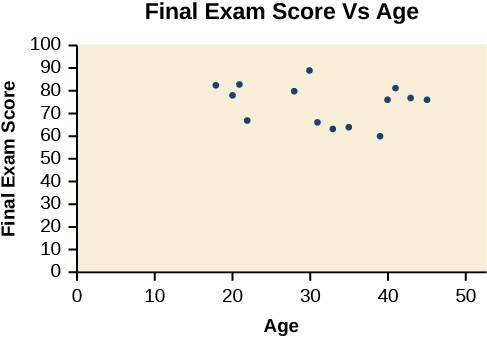Figure 1. A scatter plot of age and final exam score variables

Notice this scatter plot does not indicate a linear relationship. The points do not appear to follow a trend. In other words, there does not appear to be a relationship between the age of the student and the score on the final exam.

Example 1: Using a Scatter Plot to Investigate Cricket Chirps

The table below shows the number of cricket chirps in 15 seconds, for several different air temperatures, in degrees Fahrenheit. Plot this data, and determine whether the data appears to be linearly related.

 Chirps 44 35 20.4 33 31 35 18.5 37 26 Temperature 80.5 70.5 57 66 68 72 52 73.5 53

Solution

Plotting this data suggests that there may be a trend. We can see from the trend in the data that the number of chirps increases as the temperature increases. The trend appears to be roughly linear, though certainly not perfectly so.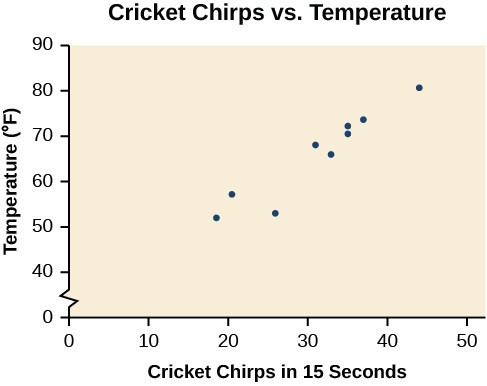Figure 2

Finding the Line of Best Fit

Once we recognize a need for a linear function to model the data in “Draw and interpret scatter plots,” the natural follow-up question is “what is that linear function?” One way to approximate our linear function is to sketch the line that seems to best fit the data. Then we can extend the line until we can verify the y-intercept. We can approximate the slope of the line by extending it until we can estimate the $\frac{\text{rise}}{\text{run}}$.

Example 2: Finding a Line of Best Fit

Find a linear function that fits the data in the table below by “eyeballing” a line that seems to fit.

 Chirps 44 35 20.4 33 31 35 18.5 37 26 Temperature 80.5 70.5 57 66 68 72 52 73.5 53

Solution

On a graph, we could try sketching a line.

Using the starting and ending points of our hand drawn line, points (0, 30) and (50, 90), this graph has a slope of

$m=\frac{60}{50}=1.2$

and a y-intercept at 30. This gives an equation of

$T\left(c\right)=1.2c+30$

where c is the number of chirps in 15 seconds, and T(c) is the temperature in degrees Fahrenheit. The resulting equation is represented in the graph below.

Analysis of the Solution

This linear equation can then be used to approximate answers to various questions we might ask about the trend.

Recognizing Interpolation or Extrapolation

While the data for most examples does not fall perfectly on the line, the equation is our best guess as to how the relationship will behave outside of the values for which we have data. We use a process known as interpolation when we predict a value inside the domain and range of the data. The process of extrapolation is used when we predict a value outside the domain and range of the data.

The graph below compares the two processes for the cricket-chirp data addressed in Example 2. We can see that interpolation would occur if we used our model to predict temperature when the values for chirps are between 18.5 and 44. Extrapolation would occur if we used our model to predict temperature when the values for chirps are less than 18.5 or greater than 44.

There is a difference between making predictions inside the domain and range of values for which we have data and outside that domain and range. Predicting a value outside of the domain and range has its limitations. When our model no longer applies after a certain point, it is sometimes called model breakdown. For example, predicting a cost function for a period of two years may involve examining the data where the input is the time in years and the output is the cost. But if we try to extrapolate a cost when x = 50, that is in 50 years, the model would not apply because we could not account for factors fifty years in the future.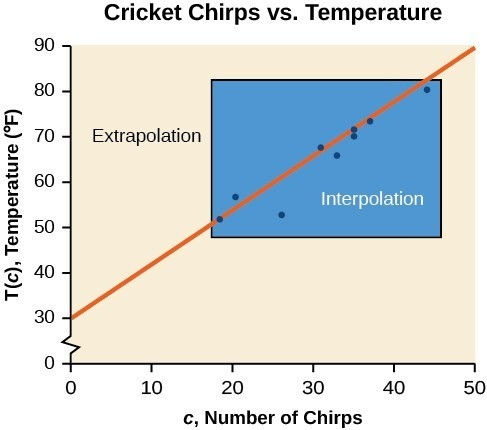Figure 4. Interpolation occurs within the domain and range of the provided data whereas extrapolation occurs outside.

A General Note: Interpolation and Extrapolation

Different methods of making predictions are used to analyze data.

• The method of interpolation involves predicting a value inside the domain and/or range of the data.
• The method of extrapolation involves predicting a value outside the domain and/or range of the data.
• Model breakdown occurs at the point when the model no longer applies.

Example 3: Understanding Interpolation and Extrapolation

 Chirps 44 35 20.4 33 31 35 18.5 37 26 Temperature 80.5 70.5 57 66 68 72 52 73.5 53

Use the cricket data above to answer the following questions:

1. Would predicting the temperature when crickets are chirping 30 times in 15 seconds be interpolation or extrapolation? Make the prediction, and discuss whether it is reasonable.
2. Would predicting the number of chirps crickets will make at 40 degrees be interpolation or extrapolation? Make the prediction, and discuss whether it is reasonable.

Solution

1. The number of chirps in the data provided varied from 18.5 to 44. A prediction at 30 chirps per 15 seconds is inside the domain of our data, so would be interpolation. Using our model:
$\begin{cases}T\left(30\right)=30+1.2\left(30\right)\hfill \\ \text{ }=66\text{degrees}\hfill \end{cases}$

Based on the data we have, this value seems reasonable.

2. The temperature values varied from 52 to 80.5. Predicting the number of chirps at 40 degrees is extrapolation because 40 is outside the range of our data. Using our model:
$\begin{cases}40=30+1.2c\hfill \\ 10=1.2c\hfill \\ c\approx 8.33\hfill \end{cases}$

We can compare the regions of interpolation and extrapolation using the graph below.

Analysis of the Solution

Our model predicts the crickets would chirp 8.33 times in 15 seconds. While this might be possible, we have no reason to believe our model is valid outside the domain and range. In fact, generally crickets stop chirping altogether below around 50 degrees.

Try It 1

According to the data from the table in Example 3, what temperature can we predict it is if we counted 20 chirps in 15 seconds?

Solution

Finding the Line of Best Fit Using a Graphing Utility

While eyeballing a line works reasonably well, there are statistical techniques for fitting a line to data that minimize the differences between the line and data values. One such technique is called least squares regression and can be computed by many graphing calculators, spreadsheet software, statistical software, and many web-based calculators. Least squares regression is one means to determine the line that best fits the data, and here we will refer to this method as linear regression.

How To: Given data of input and corresponding outputs from a linear function, find the best fit line using linear regression.

1. Enter the input in List 1 (L1).
2. Enter the output in List 2 (L2).
3. On a graphing utility, select Linear Regression (LinReg).

Example 4: Finding a Least Squares Regression Line

Find the least squares regression line using the cricket-chirp data in the table below.

 Chirps 44 35 20.4 33 31 35 18.5 37 26 Temperature 80.5 70.5 57 66 68 72 52 73.5 53

Solution

1. Enter the input (chirps) in List 1 (L1).
2. Enter the output (temperature) in List 2 (L2). See the table below.
 L1 44 35 20.4 33 31 35 18.5 37 26 L2 80.5 70.5 57 66 68 72 52 73.5 53
3. On a graphing utility, select Linear Regression (LinReg). Using the cricket chirp data from earlier, with technology we obtain the equation:
$T\left(c\right)=30.281+1.143c$

Analysis of the Solution

Notice that this line is quite similar to the equation we “eyeballed” but should fit the data better. Notice also that using this equation would change our prediction for the temperature when hearing 30 chirps in 15 seconds from 66 degrees to:

$\begin{cases}T\left(30\right)=30.281+1.143\left(30\right)\hfill \\ \text{ }=64.571\hfill \\ \text{ }\approx 64.6\text{ degrees}\hfill \end{cases}$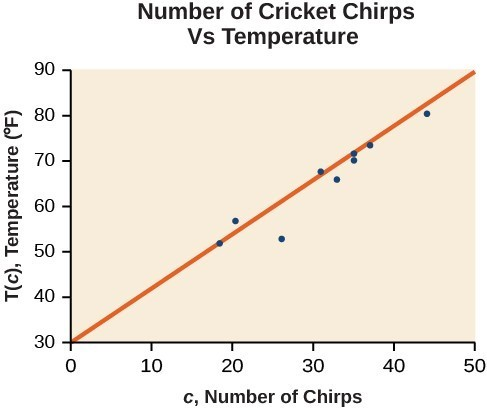Figure 6

The graph of the scatter plot with the least squares regression line is shown in below.

Q & A

Will there ever be a case where two different lines will serve as the best fit for the data?

No. There is only one best fit line.

Distinguishing Between Linear and Non-Linear Models

As we saw in “Find the line of best fit” with the cricket-chirp model, some data exhibit strong linear trends, but other data, like the final exam scores plotted by age, are clearly nonlinear. Most calculators and computer software can also provide us with the correlation coefficient, which is a measure of how closely the line fits the data. Many graphing calculators require the user to turn a “diagnostic on” selection to find the correlation coefficient, which mathematicians label as r. The correlation coefficient provides an easy way to get an idea of how close to a line the data falls.

We should compute the correlation coefficient only for data that follows a linear pattern or to determine the degree to which a data set is linear. If the data exhibits a nonlinear pattern, the correlation coefficient for a linear regression is meaningless. To get a sense for the relationship between the value of r and the graph of the data, the image below shows some large data sets with their correlation coefficients. Remember, for all plots, the horizontal axis shows the input and the vertical axis shows the output.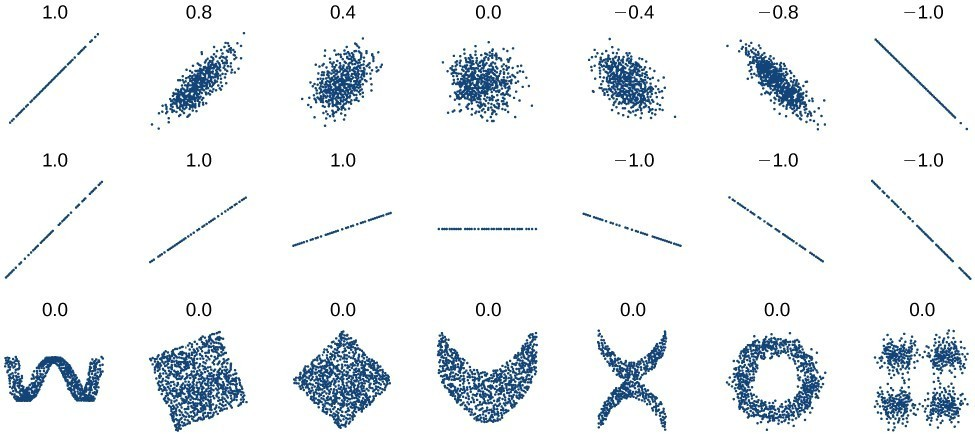Figure 7. Plotted data and related correlation coefficients. (credit: “DenisBoigelot,” Wikimedia Commons)

A General Note: Correlation Coefficient

The correlation coefficient is a value, r, between –1 and 1.

• r > 0 suggests a positive (increasing) relationship
• r < 0 suggests a negative (decreasing) relationship
• The closer the value is to 0, the more scattered the data.
• The closer the value is to 1 or –1, the less scattered the data is.

Example 5: Finding a Correlation Coefficient

Calculate the correlation coefficient for cricket-chirp data in the table below.

 Chirps 44 35 20.4 33 31 35 18.5 37 26 Temperature 80.5 70.5 57 66 68 72 52 73.5 53

Solution

Because the data appear to follow a linear pattern, we can use technology to calculate r. Enter the inputs and corresponding outputs and select the Linear Regression. The calculator will also provide you with the correlation coefficient, = 0.9509. This value is very close to 1, which suggests a strong increasing linear relationship.

Note: For some calculators, the Diagnostics must be turned “on” in order to get the correlation coefficient when linear regression is performed: [2nd]>>[alpha][x–1], then scroll to DIAGNOSTICSON.

Predicting with a Regression Line

Once we determine that a set of data is linear using the correlation coefficient, we can use the regression line to make predictions. As we learned previously, a regression line is a line that is closest to the data in the scatter plot, which means that only one such line is a best fit for the data.

Example 6: Using a Regression Line to Make Predictions

Gasoline consumption in the United States has been steadily increasing. Consumption data from 1994 to 2004 is shown in the table below. Determine whether the trend is linear, and if so, find a model for the data. Use the model to predict the consumption in 2008.

 Year ’94 ’95 ’96 ’97 ’98 ’99 ’00 ’01 ’02 ’03 ’04 Consumption (billions of gallons) 113 116 118 119 123 125 126 128 131 133 136

The scatter plot of the data, including the least squares regression line, is shown in Figure 8.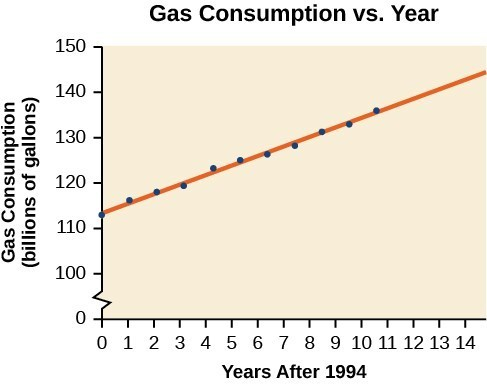Figure 8

Solution

We can introduce new input variable, t, representing years since 1994.

The least squares regression equation is:

$C\left(t\right)=113.318+2.209t$

Using technology, the correlation coefficient was calculated to be 0.9965, suggesting a very strong increasing linear trend.

Using this to predict consumption in 2008 $\left(t=14\right)$,

$\begin{cases}C\left(14\right)=113.318+2.209\left(14\right)\hfill \\ \text{ }=144.244\hfill \end{cases}$

The model predicts 144.244 billion gallons of gasoline consumption in 2008.

Try It 2

Use the model we created using technology in Example 6 to predict the gas consumption in 2011. Is this an interpolation or an extrapolation?

Solution

Key Concepts

• Scatter plots show the relationship between two sets of data.
• Scatter plots may represent linear or non-linear models.
• The line of best fit may be estimated or calculated, using a calculator or statistical software.
• Interpolation can be used to predict values inside the domain and range of the data, whereas extrapolation can be used to predict values outside the domain and range of the data.
• The correlation coefficient, r, indicates the degree of linear relationship between data.
• A regression line best fits the data.
• The least squares regression line is found by minimizing the squares of the distances of points from a line passing through the data and may be used to make predictions regarding either of the variables.

Glossary

correlation coefficient
a value, r, between –1 and 1 that indicates the degree of linear correlation of variables, or how closely a regression line fits a data set.
extrapolation
predicting a value outside the domain and range of the data
interpolation
predicting a value inside the domain and range of the data
least squares regression
a statistical technique for fitting a line to data in a way that minimizes the differences between the line and data values
model breakdown
when a model no longer applies after a certain point

Problem Set

1. Describe what it means if there is a model breakdown when using a linear model.

2. What is interpolation when using a linear model?

3. What is extrapolation when using a linear model?

4. Explain the difference between a positive and a negative correlation coefficient.

5. Explain how to interpret the absolute value of a correlation coefficient.

6. A regression was run to determine whether there is a relationship between hours of TV watched per day (x) and number of sit-ups a person can do (y). The results of the regression are given below. Use this to predict the number of sit-ups a person who watches 11 hours of TV can do.

$\begin{cases}y=ax+b\\ a=-1.341\\ b=32.234\\ r=-0.896\end{cases}$

7. A regression was run to determine whether there is a relationship between the diameter of a tree (x, in inches) and the tree’s age (y, in years). The results of the regression are given below. Use this to predict the age of a tree with diameter 10 inches.

$\begin{cases}y=ax+b\\ a=6.301\\ b=-1.044\\ r=-0.970\end{cases}$

For the following exercises, draw a scatter plot for the data provided. Does the data appear to be linearly related?

8.

 0 2 4 6 8 10 –22 –19 –15 –11 –6 –2

9.

 1 2 3 4 5 6 46 50 59 75 100 136

10.

 100 250 300 450 600 750 12 12.6 13.1 14 14.5 15.2

11.

 1 3 5 7 9 11 1 9 28 65 125 216

12. For the following data, draw a scatter plot. If we wanted to know when the population would reach 15,000, would the answer involve interpolation or extrapolation? Eyeball the line, and estimate the answer.

 Year Population 1990 11,500 1995 12,100 2000 12,700 2005 13,000 2010 13,750

13. For the following data, draw a scatter plot. If we wanted to know when the temperature would reach 28 °F, would the answer involve interpolation or extrapolation? Eyeball the line and estimate the answer.

 Temperature, °F 16 18 20 25 30 Time, seconds 46 50 54 55 62

For the following exercises, match each scatterplot with one of the four specified correlations below.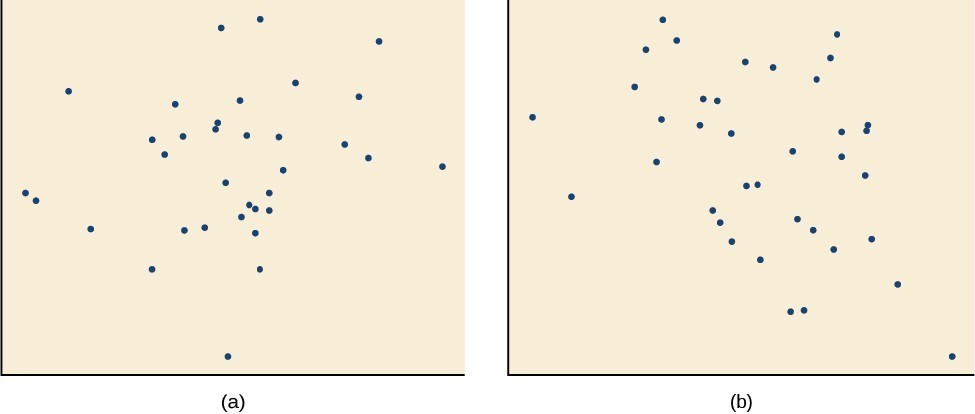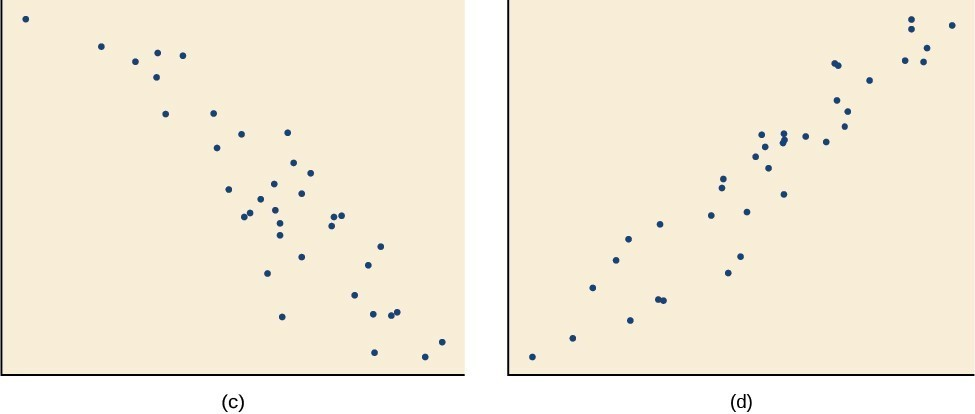14. $r=0.\text{95}$

15. $r=-0.\text{89}$

16. $r=0.26$

17. $r=-0.39$

For the following exercises, draw a best-fit line for the plotted data.

18.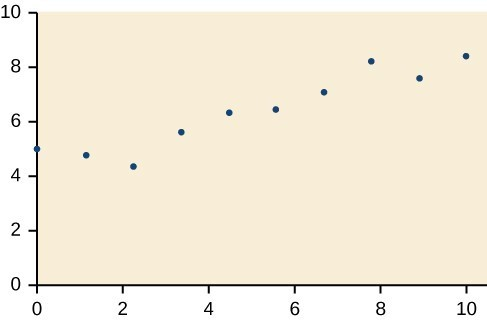19.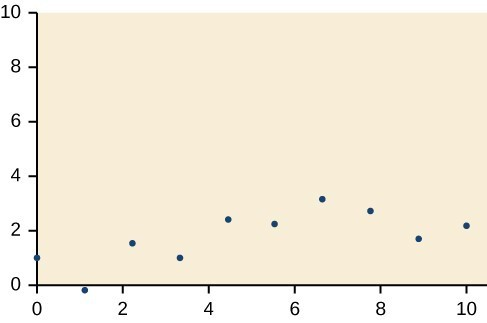20.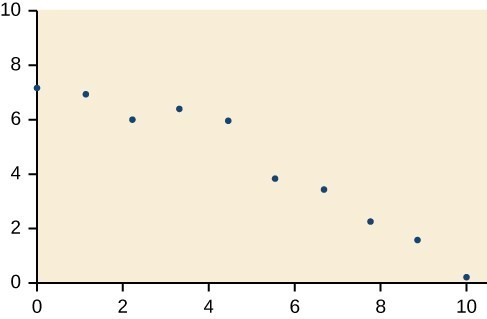21.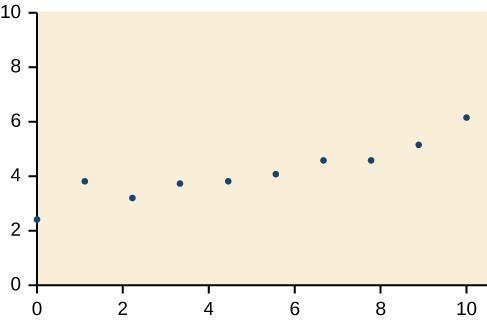22. The U.S. Census tracks the percentage of persons 25 years or older who are college graduates. That data for several years is given in the table below. Determine whether the trend appears linear. If so, and assuming the trend continues, in what year will the percentage exceed 35%?

 Year Percent Graduates 1990 21.3 1992 21.4 1994 22.2 1996 23.6 1998 24.4 2000 25.6 2002 26.7 2004 27.7 2006 28 2008 29.4

23. The U.S. import of wine (in hectoliters) for several years is given below. Determine whether the trend appears linear. If so, and assuming the trend continues, in what year will imports exceed 12,000 hectoliters?

 Year Imports 1992 2665 1994 2688 1996 3565 1998 4129 2000 4584 2002 5655 2004 6549 2006 7950 2008 8487 2009 9462

24. The table below shows the year and the number of people unemployed in a particular city for several years. Determine whether the trend appears linear. If so, and assuming the trend continues, in what year will the number of unemployed reach 5?

 Year Number Unemployed 1990 750 1992 670 1994 650 1996 605 1998 550 2000 510 2002 460 2004 420 2006 380 2008 320

For the following exercises, use each set of data to calculate the regression line using a calculator or other technology tool, and determine the correlation coefficient to 3 decimal places of accuracy.

25.

 x 8 15 26 31 56 y 23 41 53 72 103

26.

 x 5 7 10 12 15 y 4 12 17 22 24

27.

 x y x y 3 21.9 11 15.76 4 22.22 12 13.68 5 22.74 13 14.1 6 22.26 14 14.02 7 20.78 15 11.94 8 17.6 16 12.76 9 16.52 17 11.28 10 18.54 18 9.1

28.

 x y 4 44.8 5 43.1 6 38.8 7 39 8 38 9 32.7 10 30.1 11 29.3 12 27 13 25.8

29.

 x y 21 17 25 11 30 2 31 −1 40 −18 50 −40

30.

 x y 100 2000 80 1798 60 1589 55 1580 40 1390 20 1202

31.

 x y 900 70 988 80 1000 82 1010 84 1200 105 1205 108

32. Graph $f\left(x\right)=0.5x+10$ . Pick a set of 5 ordered pairs using inputs $x=\text{-2},\text{ 1},\text{ 5},\text{ 6},\text{ 9}$ and use linear regression to verify that the function is a good fit for the data.

33. Graph $f\left(x\right)=-2x - 10$. Pick a set of 5 ordered pairs using inputs $x=\text{-2},\text{ 1},\text{ 5},\text{ 6},\text{ 9}$ and use linear regression to verify the function.

For the following exercises, consider this scenario: The profit of a company decreased steadily over a ten-year span. The following ordered pairs shows dollars and the number of units sold in hundreds and the profit in thousands of over the ten-year span, (number of units sold, profit) for specific recorded years:

$\left(\text{46},\text{ 1},\text{6}00\right), \left(\text{48},\text{ 1},\text{55}0\right), \left(\text{5}0,\text{ 1},\text{5}0\text{5}\right), \left(\text{52},\text{ 1},\text{54}0\right), \left(\text{54},\text{ 1},\text{495}\right)$ .

34. Use linear regression to determine a function where the profit in thousands of dollars depends on the number of units sold in hundreds.

35. Find to the nearest tenth and interpret the x-intercept.

36. Find to the nearest tenth and interpret the y-intercept.

For the following exercises, consider this scenario: The population of a city increased steadily over a ten-year span. The following ordered pairs shows the population and the year over the ten-year span, (population, year) for specific recorded years:

(2500, 2000), (2650, 2001), (3000, 2003), (3500, 2006), (4200, 2010)

37. Use linear regression to determine a function y, where the year depends on the population. Round to three decimal places of accuracy.

38. Predict when the population will hit 8,000.

For the following exercises, consider this scenario: The profit of a company increased steadily over a ten-year span. The following ordered pairs show the number of units sold in hundreds and the profit in thousands of over the ten year span, (number of units sold, profit) for specific recorded years:

(46, 250),(48, 305),(50, 350),(52, 390),(54, 410)

39. Use linear regression to determine a function y, where the profit in thousands of dollars depends on the number of units sold in hundreds.

40. Predict when the profit will exceed one million dollars.

For the following exercises, consider this scenario: The profit of a company decreased steadily over a ten-year span. The following ordered pairs show dollars and the number of units sold in hundreds and the profit in thousands of over the ten-year span (number of units sold, profit) for specific recorded years:

(46, 250), (48, 225), (50, 205), (52, 180), (54, 165).

41. Use linear regression to determine a function y, where the profit in thousands of dollars depends on the number of units sold in hundreds.

42. Predict when the profit will dip below the $25,000 threshold. 43. Determine whether the algebraic equation is linear. $2x+3y=7$ 44. Determine whether the algebraic equation is linear. $6{x}^{2}-y=5$ 45. Determine whether the function is increasing or decreasing. $f\left(x\right)=7x - 2$ 46. Determine whether the function is increasing or decreasing. $g\left(x\right)=-x+2$ 47. Given each set of information, find a linear equation that satisfies the given conditions, if possible. Passes through $\left(\text{7},\text{5}\right)$ and $\left(\text{3},\text{17}\right)$ 48. Given each set of information, find a linear equation that satisfies the given conditions, if possible. x-intercept at $\left(\text{6},0\right)$ and y-intercept at $\left(0,\text{1}0\right)$ 49. Find the slope of the line shown in the line graph.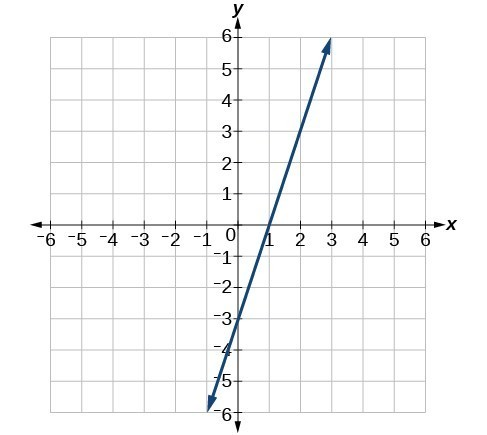50. Find the slope of the line graphed.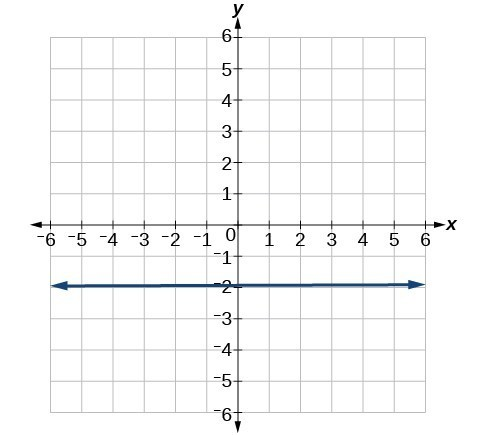51. Write an equation in slope-intercept form for the line shown.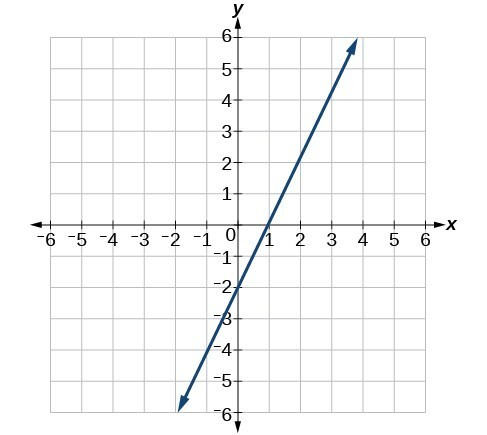52. Does the following table represent a linear function? If so, find the linear equation that models the data.  x –4 0 2 10 g(x) 18 –2 –12 –52 53. Does the following table represent a linear function? If so, find the linear equation that models the data.  x 6 8 12 26 g(x) –8 –12 –18 –46 54. On June 1st, a company has$4,000,000 profit. If the company then loses 150,000 dollars per day thereafter in the month of June, what is the company’s profit nth day after June 1st?

For the following exercises, determine whether the lines given by the equations below are parallel, perpendicular, or neither parallel nor perpendicular:

55. $\begin{cases}2x - 6y=12\hfill \\ -x+3y=1\hfill \end{cases}$

56. $\begin{cases} y=\frac{1}{3}x - 2\hfill \\ 3x+y=-9\hfill \end{cases}$

For the following exercises, find the x– and y– intercepts of the given equation

57. $7x+9y=-63$

58. $f\left(x\right)=2x - 1$

For the following exercises, use the descriptions of the pairs of lines to find the slopes of Line 1 and Line 2. Is each pair of lines parallel, perpendicular, or neither?

59. Line 1: Passes through $\left(5,11\right)$ and $\left(10,1\right)$
Line 2: Passes through $\left(-1,3\right)$ and $\left(-5,11\right)$

60. Line 1: Passes through $\left(8,-10\right)$ and $\left(0,-26\right)$
Line 2: Passes through $\left(2,5\right)$ and $\left(4,4\right)$

61. Write an equation for a line perpendicular to $f\left(x\right)=5x - 1$ and passing through the point (5, 20).

62. Find the equation of a line with a y- intercept of $\left(0,\text{ }2\right)$ and slope $-\frac{1}{2}$.

63. Sketch a graph of the linear function $f\left(t\right)=2t - 5$.

64. Find the point of intersection for the 2 linear functions: $\begin{cases}x=y+6\\ 2x-y=13\end{cases}$

65. A car rental company offers two plans for renting a car.

Plan A: 25 dollars per day and 10 cents per mile
Plan B: 50 dollars per day with free unlimited mileage

How many miles would you need to drive for plan B to save you money?

66. Find the area of a triangle bounded by the y axis, the line $f\left(x\right)=10 - 2x$ , and the line perpendicular to f that passes through the origin.

67. A town’s population increases at a constant rate. In 2010 the population was 55,000. By 2012 the population had increased to 76,000. If this trend continues, predict the population in 2016.

68. The number of people afflicted with the common cold in the winter months dropped steadily by 50 each year since 2004 until 2010. In 2004, 875 people were inflicted. Find the linear function that models the number of people afflicted with the common cold C as a function of the year, t. When will no one be afflicted?

For the following exercises, use the graph in Figure 1 showing the profit, y, in thousands of dollars, of a company in a given year, x, where x represents years since 1980.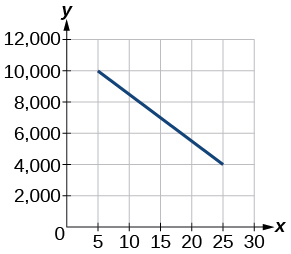Figure 1

69. Find the linear function y, where y depends on x, the number of years since 1980.

70. Find and interpret the y-intercept.

For the following exercise, consider this scenario: In 2004, a school population was 1,700. By 2012 the population had grown to 2,500.

71. Assume the population is changing linearly.
How much did the population grow between the year 2004 and 2012?
What is the average population growth per year?
Find an equation for the population, P, of the school t years after 2004.

For the following exercises, consider this scenario: In 2000, the moose population in a park was measured to be 6,500. By 2010, the population was measured to be 12,500. Assume the population continues to change linearly.

72. Find a formula for the moose population, P.

73. What does your model predict the moose population to be in 2020?

For the following exercises, consider this scenario: The median home values in subdivisions Pima Central and East Valley (adjusted for inflation) are shown in the table below. Assume that the house values are changing linearly.

Year Pima Central East Valley
1970 32,000 120,250
2010 85,000 150,000

74. In which subdivision have home values increased at a higher rate?

75. If these trends were to continue, what would be the median home value in Pima Central in 2015?

76. Draw a scatter plot for the data in the table below. Then determine whether the data appears to be linearly related.

 0 2 4 6 8 10 –105 –50 1 55 105 160

77. Draw a scatter plot for the data in the table below. If we wanted to know when the population would reach 15,000, would the answer involve interpolation or extrapolation?

 Year Population 1990 5,600 1995 5,950 2000 6,300 2005 6,600 2010 6,900

78. Eight students were asked to estimate their score on a 10-point quiz. Their estimated and actual scores are given in the table below. Plot the points, then sketch a line that fits the data.

 Predicted Actual 6 6 7 7 7 8 8 8 7 9 9 10 10 10 10 9

79. Draw a best-fit line for the plotted data.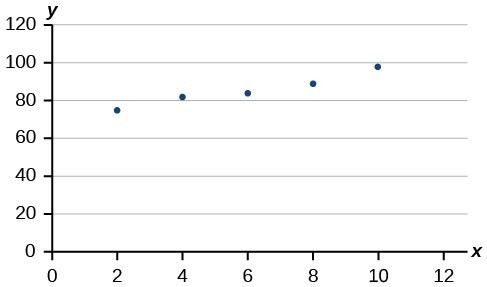For the following exercises, consider the data in the table below, which shows the percent of unemployed in a city of people 25 years or older who are college graduates is given below, by year.

 Year Percent Graduates 2000 6.5 2002 7.0 2005 7.4 2007 8.2 2010 9.0

80. Determine whether the trend appears to be linear. If so, and assuming the trend continues, find a linear regression model to predict the percent of unemployed in a given year to three decimal places.

81. In what year will the percentage exceed 12%?

82. Based on the set of data given below, calculate the regression line using a calculator or other technology tool, and determine the correlation coefficient to three decimal places.

 x 17 20 23 26 29 y 15 25 31 37 40

83. Based on the set of data given below, calculate the regression line using a calculator or other technology tool, and determine the correlation coefficient to three decimal places.

 x 10 12 15 18 20 y 36 34 30 28 22

For the following exercises, consider this scenario: The population of a city increased steadily over a ten-year span. The following ordered pairs show the population and the year over the ten-year span (population, year) for specific recorded years:

(3,600, 2000); (4,000, 2001); (4,700, 2003); (6,000, 2006)

84. Use linear regression to determine a function y, where the year depends on the population, to three decimal places of accuracy.

85. Predict when the population will hit 12,000.

86. What is the correlation coefficient for this model to three decimal places of accuracy?

87. According to the model, what is the population in 2014?

1. Selected data from http://classic.globe.gov/fsl/scientistsblog/2007/10/. Retrieved Aug 3, 2010
2. Technically, the method minimizes the sum of the squared differences in the vertical direction between the line and the data values.
3. For example, http://www.shodor.org/unchem/math/lls/leastsq.html
4. http://www.bts.gov/publications/national_transportation_statistics/2005/html/table_04_10.html
5. http://www.census.gov/hhes/socdemo/education/data/cps/historical/index.html. Accessed 5/1/2014.